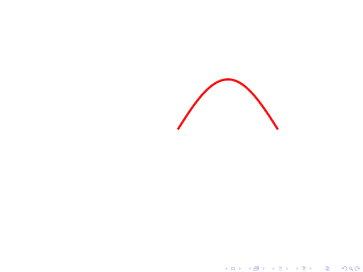## Robert Harlander

 phone: +49-241-80-27045 fax: +49-241-80-22187 harlander(at)physik.rwth-aachen.de Büro: 28A414, Campus Melaten

Home

Brief CV

Research

Teaching

Outreach

Conferences

– Software –

Press

collaborations:

LHCHWG

LHC and Philosophy

P3H

DFG RTG

[Up: aximate]

## curve

 aximate also allows to move curves from one shape to another. The curves are parametrized as (x(\$t),y(\$t)), where the parameter \$t ∈ [0,1]. A simple example is ```curve({"function" => [['100*\$t','50*sin(pi*\$t)'], ['100*\$t','-50*sin(pi*\$t)']]}, {"function" => ['\$t','\$t**2']}); ``` Note that you can use evolution functions here as well. A curve in aximate is actually a polygon of many points. The number of these points is determined by the option npoints.## Options

```	"function" => [['100*sin(2*pi*\$t)',
'100*cos(2*pi*\$t)'],
['50*cos(8*pi*\$t)',
'50*sin(8*pi*\$t)']],
"npoints" => 100,
"color" => "Red",
"width" => []
```

main
aximate:curve (Robert Harlander)